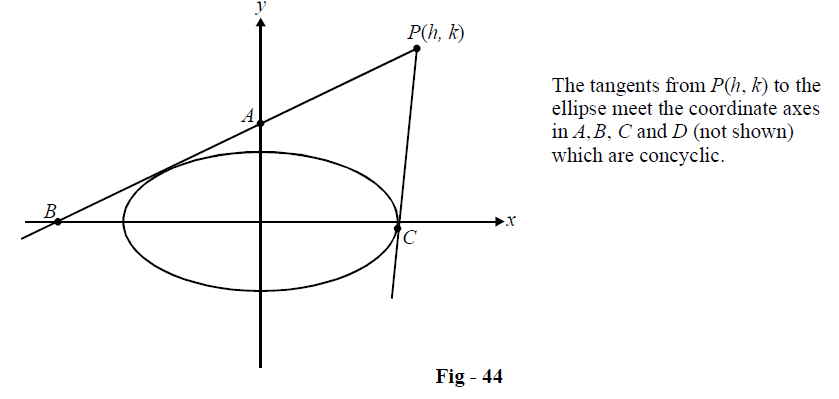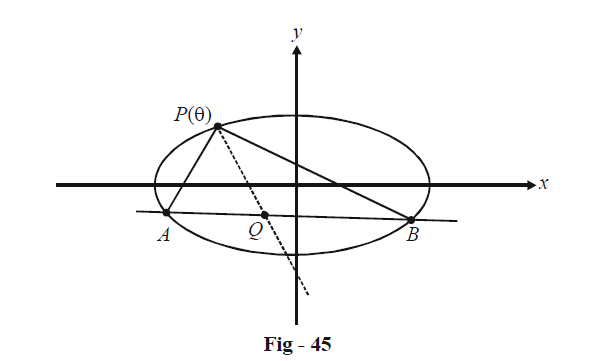# Ellipses Set-4

Go back to  'SOLVED EXAMPLES'

Example - 7

Find the locus of the point P such that tangents drawn from it to the ellipse $$\frac{{{x^2}}}{{{a^2}}} + \frac{{{y^2}}}{{{b^2}}} = 1$$ meet the coordinates axes in concyclic points.

Solution:The pair of tangents PA and PC has the joint equation

\begin{align}&\qquad~~{J_1}\quad :\quad {T^2}(h,\,k) = S(x,y)S(h,k)\\ &\Rightarrow \quad {J_1} \quad:\quad \left( {\frac{{{x^2}}}{{{a^2}}} + \frac{{{y^2}}}{{{b^2}}} - 1} \right)\left( {\frac{{{h^2}}}{{{a^2}}} + \frac{{{k^2}}}{{{b^2}}} - 1} \right) - {\left( {\frac{{hx}}{{{a^2}}} + \frac{{ky}}{{{b^2}}} - 1} \right)^2} = 0\end{align}

The coordinate axes has the joint equation

${{J}_{2}}:xy=0$

We can treat J1 and J2 as two curves, which intersect in four different points A, B, C, D. Any second degree curve through these four points can be written in terms of a parameter $$\lambda$$ as

${J_1} + \lambda {J_2} = 0$

We now simply find that $$\lambda$$ for which this represents a circle, since A, B, C, D are given to be concyclic.

$\Rightarrow \left( \frac{{{x}^{2}}}{{{a}^{2}}}+\frac{{{y}^{2}}}{{{b}^{2}}}-1 \right)\left( \frac{{{h}^{2}}}{{{a}^{2}}}+\frac{{{k}^{2}}}{{{b}^{2}}}-1 \right)-{{\left( \frac{hx}{{{a}^{2}}}+\frac{ky}{{{b}^{2}}}-1 \right)}^{2}}+\lambda xy=0$

This represents a circle if

\begin{align}&{\bf{Coeff}}{\bf{. of \;}}{x^{\bf{2}}}{\bf{ = Coeff}}{\bf{. of\; }}{y^{\bf{2}}} \qquad \Rightarrow \quad \frac{{{k^2}}}{{{a^2}{b^2}}} - \frac{1}{{{a^2}}} = \frac{{{h^2}}}{{{a^2}{b^2}}} - \frac{1}{{{b^2}}}\\&\qquad\qquad\qquad\qquad\qquad\qquad\qquad{\rm{ \;}} \Rightarrow {h^2} - {k^2} = {a^2} - {b^2}\quad\quad\quad...\left( 1 \right)\\&{\bf{Coeff}}{\bf{. of \;}}xy{\bf{ = 0}} \qquad\qquad \qquad\;\;\Rightarrow \lambda = \frac{{2hk}}{{{a^2}{b^2}}}\qquad\qquad\qquad\;\;...\left( 2 \right)\end{align}

(1) itself gives the locus of P(h, k) as

${x^2} - {y^2} = {a^2} - {b^2}$

Example - 8

Through any arbitrary fixed point $$P(\theta )$$ on the ellipse $$\frac{{{x^2}}}{{{a^2}}} + \frac{{{y^2}}}{{{b^2}}} = 1,$$ chords at right angles are drawn, such that the line joining the extremities of these chords meets the normal through P at the point Q. Prove that Q is fixed for all such chords.

Solution:We can assume the eccentric angles of A and B as $${\theta _1}\;and\;{\theta _2}$$

The normal at P has the equation:

$PQ:ax\sec \theta - by\,{\rm{cosec }}\theta = {a^2} - {b^2}\quad\quad\quad...\left( 1 \right)$

The chord AB has the equation

$AB:\frac{x}{a}\cos \left( {\frac{{{\theta _1} + {\theta _2}}}{2}} \right) + \frac{y}{b}\sin \left( {\frac{{{\theta _1} + {\theta _2}}}{2}} \right) = \cos \left( {\frac{{{\theta _1} - {\theta _2}}}{2}} \right)\quad\quad\quad...\left( 2 \right)$

Also, since $$PA \bot PB,$$ we have

\begin{align}&\qquad\underbrace {\frac{{ - b\cos \left( {\frac{{\theta + {\theta _1}}}{2}} \right)}}{{a\sin \left( {\frac{{\theta + {\theta _1}}}{2}} \right)}}}_{{\rm{slope of chord }}PA}\,\,\,\, \times \,\,\,\underbrace {\frac{{ - b\cos \left( {\frac{{\theta + {\theta _2}}}{2}} \right)}}{{a\sin \left( {\frac{{\theta + {\theta _1}}}{2}} \right)}}}_{{\rm{slope of chord }}PB} = - 1\\\\& \Rightarrow\quad {a^2}\sin \left( {\frac{{\theta + {\theta _1}}}{2}} \right)\sin \left( {\frac{{\theta + {\theta _2}}}{2}} \right) + {b^2}\cos \left( {\frac{{\theta + {\theta _1}}}{2}} \right)\cos \left( {\frac{{\theta + {\theta _2}}}{2}} \right) = 0\end{align}

Using trigonometric formulae, this expression can be rearranged to

$\cos \left( {\theta + \frac{{{\theta _1} + {\theta _2}}}{2}} \right) = \frac{{{a^2} + {b^2}}}{{{a^2} - {b^2}}}\cos \left( {\frac{{{\theta _1} - {\theta _2}}}{2}} \right)\quad\qquad\qquad...\left( 3 \right)$

From (2) and (3), we have

$AB:\frac{x}{a}\cos \left( {\frac{{{\theta _1} + {\theta _2}}}{2}} \right) + \frac{y}{b}\sin \left( {\frac{{{\theta _1} + {\theta _2}}}{2}} \right) = \frac{{{a^2} - {b^2}}}{{{a^2} + {b^2}}}\cos \left( {\theta + \frac{{{\theta _1} + {\theta _2}}}{2}} \right)\quad\quad\quad...\left( 4 \right)$

The point Q can now be obtained as the intersection of the lines represented by (1) and (4). Let us write them as a system and solve for Q using the Cramer’s rule:

\begin{align}&PQ:\quad (a\sec \theta )x + ( - b\,{\rm{cosec }}\theta )y + ({b^2} - {a^2}) = 0\\& AB:\quad (b\cos \alpha )x + (a\sin \alpha )y - \frac{{ab({a^2} - {b^2})}}{{{a^2} + {b^2}}}\cos (\theta + \alpha ) = 0\end{align}

where $$\alpha = \frac{{{\theta _1} + {\theta _2}}}{2}$$ has been substituted for convenience.

We now have

$\frac{x}{{\frac{{a{b^2}({a^2} - {b^2})}}{{{a^2} + {b^2}}}{\rm{cosec }}\theta \cos (\theta + \alpha ) - a\sin \alpha ({b^2} - {a^2})}} = \frac{y}{{b({b^2} - {a^2})\cos \alpha + \frac{{{a^2}b({a^2} - {b^2})}}{{{a^2} + {b^2}}}\sec \theta \cos (\theta + \alpha )}}$

$= \frac{1}{{{a^2}\sec \theta \sin \alpha + {b^2}{\rm{cosec }}\theta \cos \alpha }}$

\begin{align} &\quad \Rightarrow\qquad x=\frac{\frac{a{{b}^{2}}({{a}^{2}}-{{b}^{2}})}{{{a}^{2}}+{{b}^{2}}}\cos (\theta +\alpha )-a\sin \theta \sin \alpha ({{b}^{2}}-{{a}^{2}})}{\sin \theta ({{a}^{2}}\sec \theta \sin \alpha +{{b}^{2}}\text{cosec }\theta \cos \alpha )} \\\\ &\qquad\qquad~~~~ \text{ }=\frac{a({{a}^{2}}-{{b}^{2}})}{{{a}^{2}}+{{b}^{2}}}\cos \theta ~~~~~~~~~~~...\left( 5 \right) \\\\& and\quad\quad~~~~\text{ }y=\frac{b({{b}^{2}}-{{a}^{2}})\cos \alpha \cos \theta +\frac{{{a}^{2}}b({{a}^{2}}-{{b}^{2}})}{{{a}^{2}}+{{b}^{2}}}\cos (\theta +\alpha )}{\cos \theta ({{a}^{2}}\sec \theta \sin \alpha +{{b}^{2}}\text{cosec }\theta \cos \alpha )} \\\\ &\qquad\qquad~~~~ ~\text{ }=\frac{b({{b}^{2}}-{{a}^{2}})\sin \theta }{{{a}^{2}}+{{b}^{2}}}~~~~~~~~~~...\left( 6 \right) \\ \end{align}

Thus, the point Q, whose x and y coordinates are given by (5) and (6) respectively, can be seen to be independent of $$\alpha \;or\;\frac{{{\theta _1} + {\theta _2}}}{2}.$$ Q is the therefore fixed for such pairs of chords PA and PB and depends only on the eccentric angle of P.

Learn from the best math teachers and top your exams

• Live one on one classroom and doubt clearing
• Practice worksheets in and after class for conceptual clarity
• Personalized curriculum to keep up with school